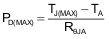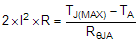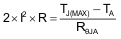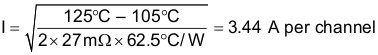SLVSCG3F January   2014  – July 2017

PRODUCTION DATA.

1. Features
2. Applications
3. Description
1.     Device Images
4. Revision History
5. Device Comparison
6. Pin Configuration and Functions
7. Specifications
8. Parameter Measurement Information
9. Detailed Description
1. 9.1 Overview
2. 9.2 Functional Block Diagram
3. 9.3 Feature Description
4. 9.4 Device Functional Modes
10. 10Application and Implementation
1. 10.1 Application Information
2. 10.2 Typical Application
1. 10.2.1 Design Requirements
2. 10.2.2 Detailed Design Procedure
3. 10.2.3 Application Curves
11. 11Power Supply Recommendations
12. 12Layout
13. 13Device and Documentation Support
14. 14Mechanical, Packaging, and Orderable Information

• DPU|14
• DPU|14

#### 10.2.2.3 Thermal Considerations

The maximum IC junction temperature must be restricted to 125°C under normal operating conditions. To calculate the maximum allowable dissipation, PD(max) for a given output current and ambient temperature, use Equation 6.

Equation 6.where

• PD(max) is the maximum allowable power dissipation
• TJ(max) is the maximum allowable junction temperature (125°C for the TPS22968)
• TA is the ambient temperature of the device
• RθJA is the junction to air thermal impedance. See theThermal Information table. This parameter is highly dependent upon board layout.

Equation 7 to Equation 10 and Equation 11 to Equation 13 show two examples to determine how to use this information correctly:

For VBIAS = 5 V, VIN = 5 V, the maximum ambient temperature with a 4-A load through each channel can be determined by using Equation 7 to Equation 10:

White Space

Equation 7. PD = I2 × R × 2 (multiplied by 2 because there are two channels)

White Space

Equation 8.White Space

Equation 9. TA = TJ(MAX) – RθJA × 2 × I2 × R

White Space

Equation 10. TA = 125°C – 62.5°C/W × 2 × (4 A)2 × 27 mΩ = 71°C

White Space

For VBIAS = 5 V, VIN = 5 V, the maximum continuous current for an ambient temperature of 85°C with the same current flowing through each channel can be determined by using Equation 11 to Equation 13:

Space

Equation 11.Space

Equation 12.Space

Equation 13.